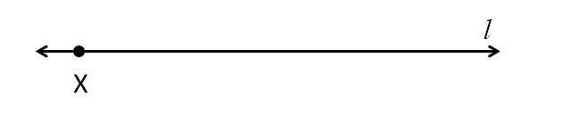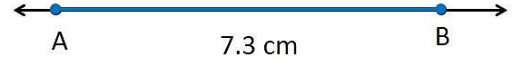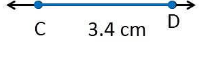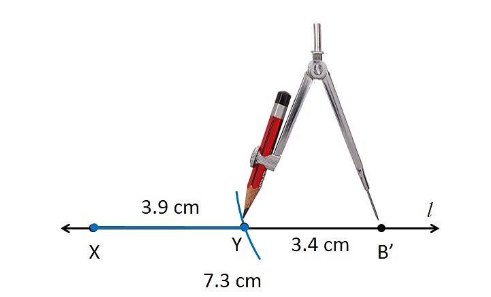Courses
Courses for Kids
Free study material
Free LIVE classes
MoreLIVE
Join Vedantu’s FREE Mastercalss

# Given $\overline {AB}$of length 7.3 cm and $\overline {CD}$ of length 3.4 cm, construct a line segment $\overline {XY}$ such that the length of $\overline {XY}$ is equal to the difference between the lengths of $\overline {AB}$ and $\overline {CD}$. Verify by measurement.Verified
360.9k+ views
Hint: We need to follow the construction steps in order.

Steps of construction:
Draw a line L and take a point X on it.
Construct XZ bar such that length $\overline {XZ}$= length of $\overline {AB}$ = 7.3 cm
Then cut off $\overline {ZY}$ = length of $\overline {CD}$= 3.4 cm
Thus the length of $\overline {XY}$ = length of $\overline {CD}$ verification : By measurement we find that length of $\overline {XY}$ = 3.9 cm = 7.3 cm – 3.4 cm = $\overline {AB}$ = $\overline {CD}$ segment $\overline {XY}$

Note:
Step 1:Step 2:Step 3:Step 4:Last updated date: 20th Sep 2023
Total views: 360.9k
Views today: 8.60k